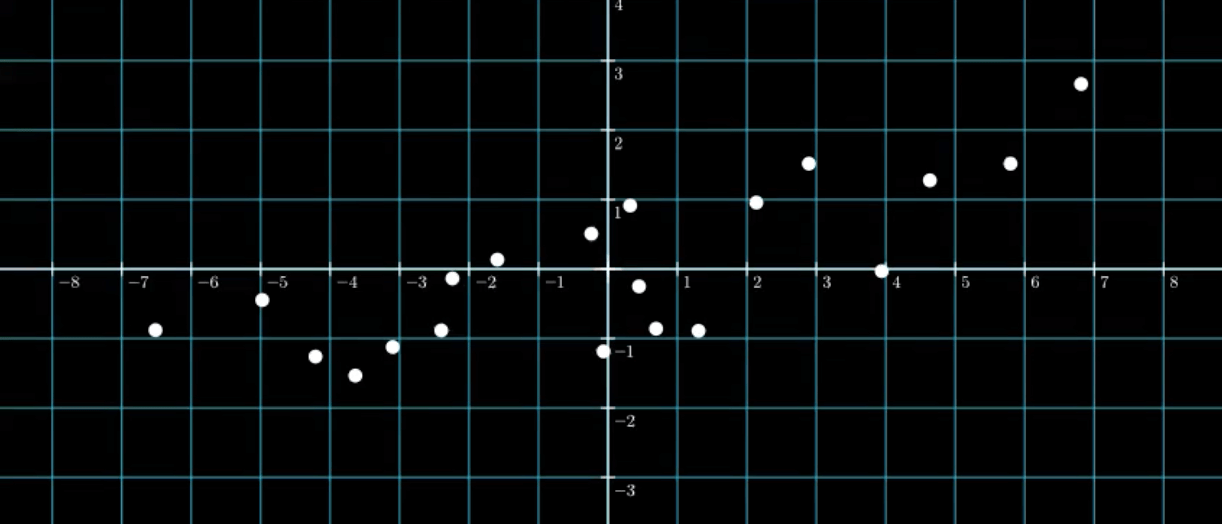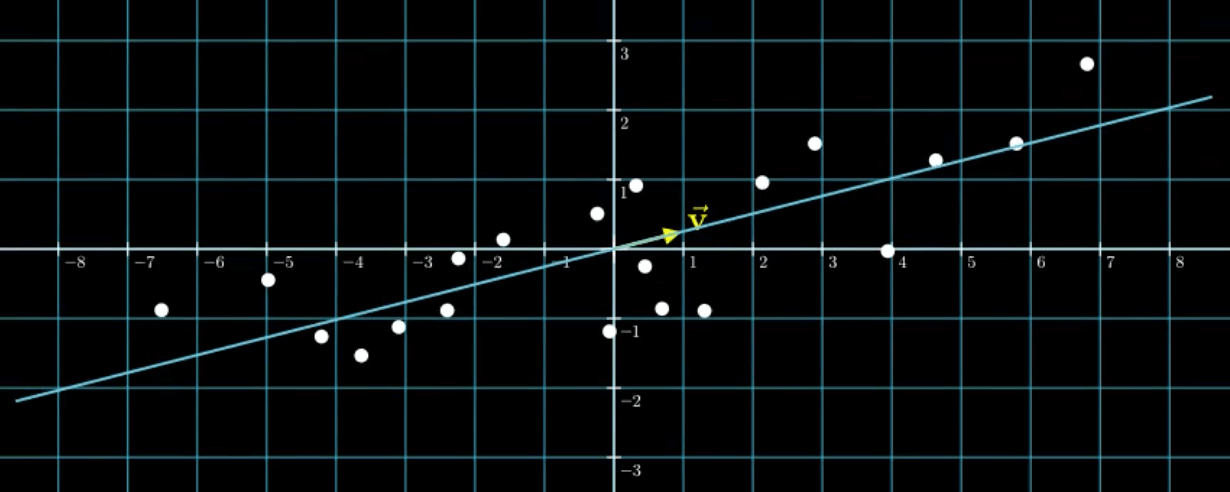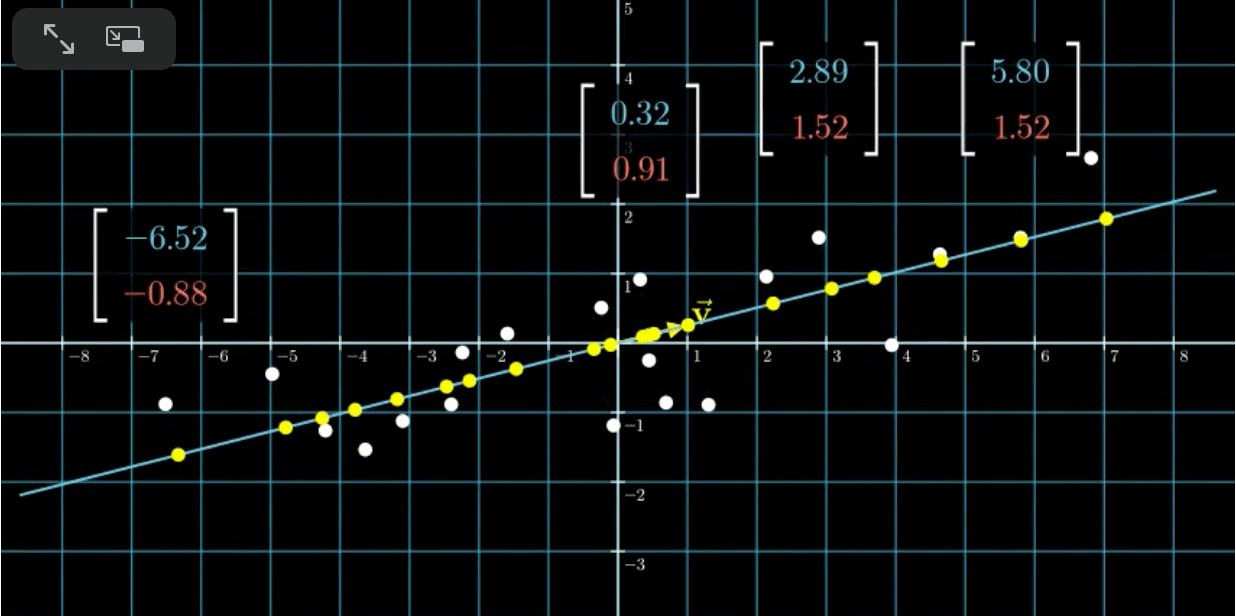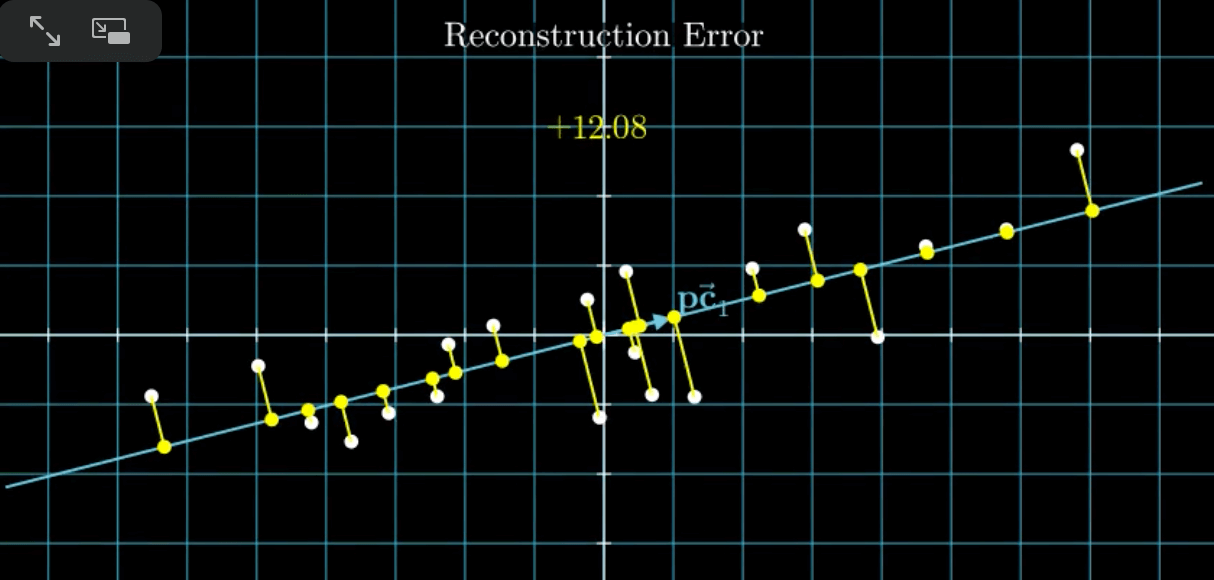# 【数学基础】第十六课：主成分分析

## 主成分分析

Posted by x-jeff on September 21, 2020

# 2.PCA的直观理解

$\begin{bmatrix} 2.89 & 1.52 \\ 0.32 & 0.91 \\ 5.8 & 1.52 \\ -6.52 & -0.88 \\ 3.94 & -0.03 \\ -4.21 & -1.26 \\ 0.45 & -0.25 \\ 2.14 & 0.96 \\ 1.3 & -0.89 \\ -4.98 & -0.45 \\ -2.4 & -0.88 \\ -3.1 & -1.12 \\ 0.69 & -0.86 \\ -1.59 & 0.13 \\ -3.64 & -1.53 \\ -0.24 & 0.51 \\ 6.81 & 2.66 \\ 4.63 & 1.28 \\ -2.24 & -0.14 \\ -0.06 & -1.19 \\ \end{bmatrix}$\begin{align} \vec{i} = \begin{bmatrix} 1\\0 \end{bmatrix}, \vec{j} = \begin{bmatrix} 0\\1 \end{bmatrix} \end{align}

$\begin{bmatrix} x\\y \end{bmatrix}=f_1 \vec{i} + f_2 \vec{j}$# 3.PCA原理剖析

1. 最大变异：降维后所得到的新K维特征L具有最大的变异（Variance）。
2. 最小错误：用K维的新特征L重新构建回N维数据能得到最小的重建错误（Reconstruction Error）。“最小错误”就是找到这样一条拟合直线，使得距离之和（即黄线的长度之和）最小，可以通过最小二乘法来确定。并且，这条拟合直线同时也满足“最大变异”。

# 4.PCA的算法流程

1. 对所有样本进行中心化：$x_i=x_i-\frac{1}{m}\sum_{j=1}^m x_j$。
2. 计算样本的协方差矩阵
3. 求出协方差矩阵的特征值及对应的特征向量
4. 将特征向量按对应特征值大小从上到下按行排列成矩阵，取前k行组成矩阵$P_{k\times n}$。
5. $Y_{k\times m}=P_{k\times n}X_{n\times m}$即为降维到k维后的数据。

# 5.参考资料